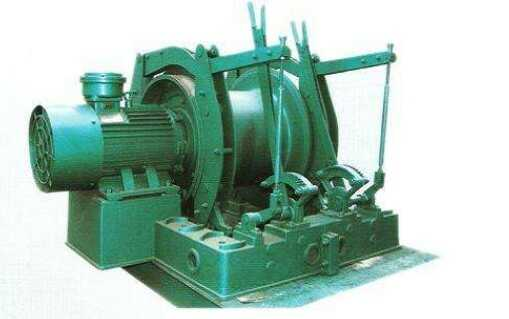# 中煤集团 - 绞车生产制造厂家

http://www.zmjiaoche.com/

# 煤矿井下调度绞车选型

调度绞车选型：
绞车的选型依据轨道下山绞车运行斜坡长度、坡度及牵引载荷的重量而定，绞车的牵引力必须大于载荷重量。
牵引载荷：
A =sin6°(Qz+ Q)×9.8×4
=0.1(3000+1500)×9.8×4
=17640N
=17.64KN
查JD-2型绞车牵引力为：20KN>17.64KN,故可选型于JD-2型绞车。
钢丝绳直径为18.5mm。
JD-2型绞车钢丝绳验算：
安全系数： m=ρh /Qj
m ——钢丝绳的安全系数
ρh ——钢丝绳破断力总和
Qj ——钢丝绳静拉力
Qj=g .(Qz+Q)n×(sinθ+f1cosθ)+g.q.L(sinθ+ f2cosθ)
=9.8×(3000+1500)×4×(sin6°+0.02cos6°) +9.8×1.2×200×(sin6°+0.2cos6°)
=21833.616N
安全系数m=ρh/Qj=166180/21833.616= 7.6>6.5
式中：
A---斜坡轨道运行时的牵引载荷
Qj ---钢丝绳静拉力
Qz---矿车自重：Qz=1500kg
Q----矿车载重：Q=3000kg
n---牵引矿车数辆：n=4辆
θ--巷道坡度：θ= 6°(sin6°=0.1 cos6°=0.99)
q---钢丝绳每米重量：q=1.2kg/m
L---钢丝绳长度：L=200m
m---钢丝绳的安全系数：
m= 6.5
ρh---钢丝绳破断力：取166.18KN
f1-矿车运动的阻力系数：(f1=0.01-0.02)取0.02
f2-钢丝绳与底板和托辊间的摩擦系数：(f2=0.15-0.2)取0.2。
经过计算，安全系数7.6>6.5符合煤矿安全规程规定，故选用φ=18.5mm的钢丝绳。 故选用JD-2型调度绞车进行运输符合要求。